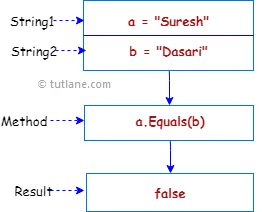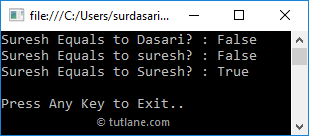# Visual Basic String Equals Method

In visual basic, the string Equals method is useful to check whether the specified two string objects are having the same value or not. If both string object values are equal, then the Equals() method will return True otherwise False. Even, if both string objects are having Null value, the string Equals() method will return True.

Following is the pictorial representation of using the string Equals() method to check whether the given string objects are equal or not in a visual basic programming language.If you observe the above diagram, we defined two strings “Suresh”, “Dasari” and checking whether both the strings are equal or not using the Equals method.

## Visual Basic String Equals Method Syntax

Following is the syntax of defining a string Equals method to check whether the given string objects are equal or not in a visual basic programming language.

Public Function Equals(ByVal a As String, ByVal b As String) As Boolean

If you observe the syntax, we will use both defined strings to check whether both the strings are equal or not using the Equals method.

## Visual Basic String Equals Method Example

Following is the example of using the string Equals() method to check whether the given strings are equal or not in a visual basic programming language.

Module Module1

Sub Main()

Dim fname As String = "Suresh"

Dim lname As String = "Dasari"

Console.WriteLine("{0} Equals to {1}? : {2}", fname, lname, fname.Equals(lname))

Dim l_name As String = "suresh"

Console.WriteLine("{0} Equals to {1}? : {2}", fname, l_name, fname.Equals(l_name))

Dim u_name As String = "Suresh"

Console.WriteLine("{0} Equals to {1}? : {2}", fname, u_name, fname.Equals(u_name))

Console.WriteLine("Press Enter Key to Exit..")

End Sub

End Module

If you observe the above example, we used a string Equals() method to check whether the specified string values are equal or not.

When we execute the above visual basic program, we will get the result like as shown below.If you observe the above result, the string Equals() method checked to know whether the specified string values are equal or not and returned a boolean value.

## Visual Basic String Equals Ignore Case

Generally, in visual basic the string Equals() method will perform case-sensitive string comparison. In case, if we want to perform case insensitive string comparison, then we need to use OrdinalIgnoreCase property along with the Equals method.

Following is the example of ignoring the case while comparing the strings in a visual basic programming language.

Module Module1

Sub Main()

Dim fname As String = "Suresh"

Dim l_name As String = "suresh"

Console.WriteLine("{0} Equals to {1}? : {2}", fname, l_name, fname.Equals(l_name, StringComparison.OrdinalIgnoreCase))

Dim u_name As String = "Suresh"

Console.WriteLine("{0} Equals to {1}? : {2}", fname, u_name, fname.Equals(u_name))

Console.WriteLine(vbLf & "Press Enter Key to Exit..")

End Sub

End Module

If you observe above example, we used “OrdinalIgnoreCase” property with the Equals() method to perform case insensitive comparison by ignoring the case of characters in a visual basic programming language.

When we execute the above visual basic program, we will get the result like as shown below.If you observe the above result, the string Equals() method has returned a True for both “Suresh” and “suresh” values by ignoring the case.

This is how we can use the Equals() method to check whether the specified string object values are equal or not based on our requirements.